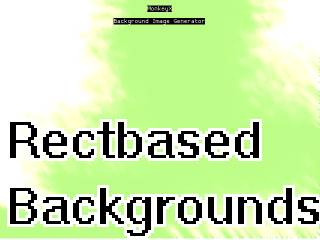-=+=- -=+=- -=+=- -=+=- -=+=- -=+=- -=+=- -=+=- -=+=- -=+=- -=+=- -=+=- -=+=- -=+=- -=+=- -=+=- -=+=- -=+=- -=+=- -=+=- -=+=- -=+=- -=+=- -=+=- -=+=- -=+=- -=+=- -=+=- -=+=- -=+=- (c) WidthPadding Industries 1987 0|302|0 -=+=- -=+=- -=+=- -=+=- -=+=- -=+=- -=+=- -=+=- -=+=- -=+=- -=+=- -=+=- -=+=- -=+=- -=+=- -=+=- -=+=- -=+=- -=+=- -=+=- -=+=- -=+=- -=+=- -=+=- -=+=- -=+=- -=+=- -=+=- -=+=- -=+=-
SoCoder -> Showcase Home -> Graphic Demos

PakzCreated : 22 May 2016
Edited : 26 May 2016
System : Cross Platform
Language : Monkey

### Grey backgrounds

Several graphical filters in action

ScreenshotsI noticed a interesting background image on the playstation 3 youtube app and decided I wanted to make something like it.

I spend a while creating this. My mother does not think the images are nice. I think they could be useable for backgrounds.

## Latest Update

Here a video of some output of a later variation on the code :
https://youtu.be/Y3YfQF8P_gs
And here the last html5
https://cromdesi.home.xs4all.nl/html5/rectbackgrounds/MonkeyGame.html

Here another variation :
https://cromdesi.home.xs4all.nl/html5/grey3backgroundex/MonkeyGame.html

I use blurring which takes a random spot in the map and then takes the pixel to the roght and bottom and bottom right and then avarages it. This is done a lot of times depending on the fuzzyness.

Sunday, 22 May 2016, 07:24
JayenkaiNice little smokey effect.
Would be handy to render those to textures, then use them in-game, but Monkey's not the best at doing that sort of thing, due to the complexities of the different targets. It's a shame, really, because doing stuff like that would be great.

Anyway, nice enough little artsy-tool thing.Sunday, 22 May 2016, 07:43
PakzIf I get some time for b3d I could use the graphic making techniques for the textures. Maybe if mx2 gets a 3d engine then I might also use this.

I keep modifying the code. Just uploaded the last version.